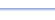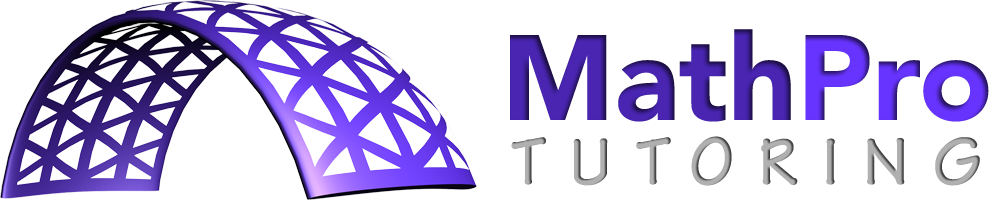Program: CONVERGE

CONVERGE

• Finds the nth term of a sequence or the sum of n terms.
• Tests to see if a sequence or series converges.  If it converges, it finds either the limit or the sum.
• Finds the limit of a function.

Useful for:

• Calculus, especially AP Calculus AB and BC
• Precalculus
• Computer Science

See full list of programsExample

Q: Does the series converge?

Solution:  Press 'Y=' and enter Y1 = 3(.6)^X
(Always use the letter X on this screen.  It's the button in the 3rd row from the top, 2nd from the left.)

Run CONVERGE.  How?

Choose 2 for 'Series'.

Start at X=1
End at X=100  (This should just be a
large number to simulate infinity.)
Increment = 1  (For a summation, n
takes on all integer values from the
bottom number to the top number.)

The program evaluates the function
at each integer value from 1 to 100,
produces cumulative totals, and stops
on the last cumulative total.  If the
last several lines are the same value,
then the series converges to that value.
If the numbers show no trend, bounce
around, or increase/decrease without
bound, then the series does not
converge (or you have not chosen a
large enough 'end' value).

Interpretation:  The series converges to 9/2.

* Note: If you want to determine if a sequence converges, choose 1 for 'Sequence'.  Then, the program will report the individual values of the function, not the totals.